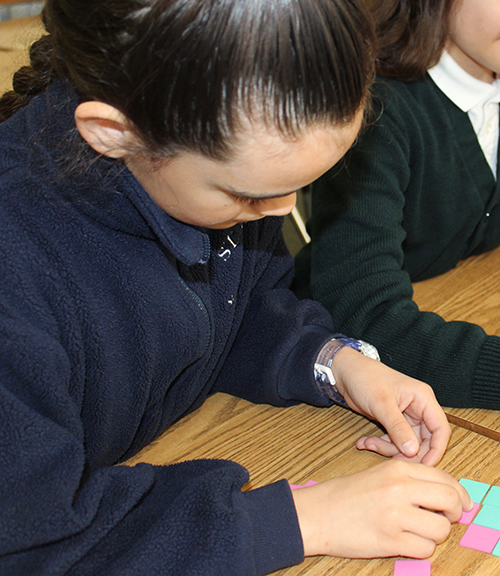• #We have transitioned into full implementation of the Math Common Core Standards in all grades, and adopted the Envision Math program for grades 2-8. 7th and 8th graders are placed on two academic tracks, including Pre-algebra and Algebra. We are excited about the growth these standards are providing for our students.

By the end of fourth grade, students will understand multi-digit multiplication and division involving multi-digit dividends. They will be able to describe and compare fraction equivalence, addition and subtraction of fractions with like denominators, and multiplication of fractions by whole numbers. They will also be able to analyze and classify geometric figures based on their properties.

By the end of fifth grade, students will develop fluency with fraction addition and subtraction, and will be introduced to fraction multiplication and division. They will extend their abilities to include division with 2-digit divisors, integrating decimal fractions into the place value system and develop understanding of operations with decimals to the thousandths. Finally, they will develop a basic understanding of volume and conversion of basic measurements.